© 2008  Rasmus ehf and Jóhann Ísak

## TrianglesLesson 1    Rules for triangles

It is common practise to label the vertices of a triangle with capital letters and the sides with small letters.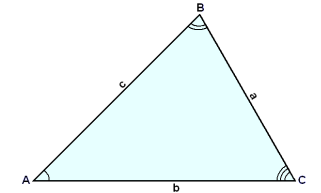It is also common practice to label the side opposite angle A with a small a, the side opposite angle B with a small b and the side opposite angle C with a small c ( see the diagram ).

The sides that form the arms of the angle A are said to be adjacent to A. The side on which the triangle stands is called the base of the triangle.

The sum of the angles of a triangle is 180° . This can easily be seen by drawing a straight line through the angle B and parallel to the side b. (see diagram).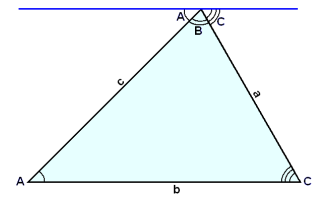The angles formed with this line are equal to A, B and C ( by the rule that alternate angles between parallel lines are equal).
Also A + B + C = 180° as together  they make a straight line .

The straight line from angle B perpendicular to the base line b is called the height of the triangle. The height is labelled h in the diagram below.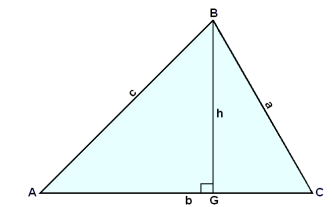You have previously learned that the area of a triangle is given by the formula.

Area F = ½×b×h

The letter G is used here to label the point where the height and the base intersect. This point is sometimes called the perpendicular projection of the point B onto the line b.

Two triangles are said to be similar if all the angles of one triangle are equal to all the angles of the other. If we want to show that two triangles are similar it is sufficient to show that two angles are equal. If two angles are equal it is obvious that the third angle in each must be equal.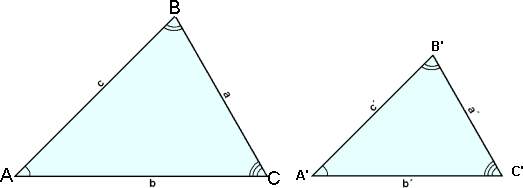The triangles in the above diagram are similar. It follows that the ratios between corresponding sides are the same.
In other words :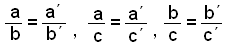and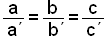We will now do some examples using these ratios.

Example 1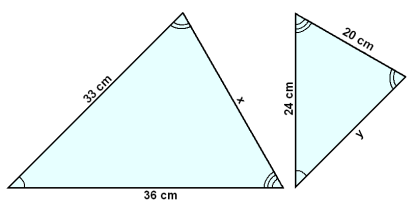The triangles in the diagram are similar with the equal angles marked in the same way. We want to calculate the length of the sides labelled x and y.

We begin by labelling the triangles so we can see more easily which sides correspond to each other.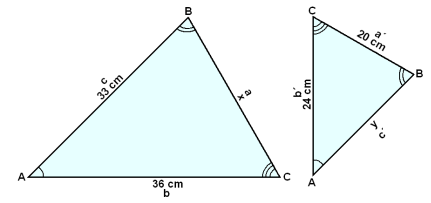We can write the following ratios:

b/c = 36/33 = 24/y = b´/c´

This means that y/24 = 33/36
and therefore y = 24×33/36 = 22 cm.

Also a/b = x/36 = 20/24 = a´/b´

Which gives us  x = 36×20/24 = 30 cm.

Another rule using proportions in triangles can be deduced.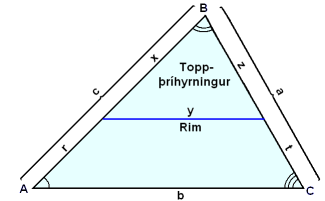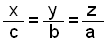We draw a straight line that cuts two sides of the triangle and is parallel to the third side. This line divides the triangle into two parts, the top part is a triangle that is similar to ABC, the original triangle. We will label the sides of this smaller triangle with the letters  x, y and z. The following is now true:

The line we have drawn divides the side c into two parts, x and r and the side a into  z and t.  Putting x + r in for c and z + t in for a in the above equation we get the following result: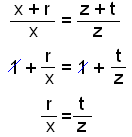We have shown that any line through two sides of a triangle and parallel to the third side divides these two sides in the same ratio.

### Example 2

Two sides of the triangle ABC, AB and BC are 30 cm in length and the third side AC, the base line,  is 42 cm. We draw a line through a point X on AB  parallel to the base and of length 14 cm. Find the length of the lines BX and AX.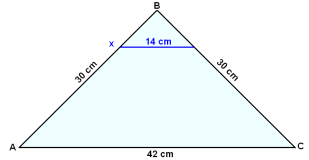14/42 = XB/30

XB = 30×14/42 = 10 cm

AX = 30 − 10 = 20 cm

In most cases if we want to find the size of the angles of a triangle we either need to draw an accurate diagram and measure the angles or use the rules of trigonometry.Equilateral.                     Isosceles.                Right angled.

In an equilateral triangle all the sides are equal and all the angles are 60°.

In an isosceles triangle two sides are the same length and two angles (the angles formed with the base line) are equal. If we know one angle in an isosceles triangle we can find the other angles. The perpendicular from the vertex to the base line (the height) in an isosceles triangle divides the triangle into two equal right angled triangles.

The sides of a right angled triangle ABC satisfy Pythagoras’ rule, that is a2 + b2 = c2 .

Also the converse is true. If Pythagoras’ rule holds then the triangle is right angled.

We can check that the third triangle in the above diagram is right angled by using if Pythagoras´ rule.

52 + (53)2 = 102

25 + 75 = 100

Note that the length of the hypotenuse (10 cm) in this triangle is twice the length of the shortest side (5 cm).
When this is the case the angles of the triangle are always 30°, 60°and 90°.

Example 3

Find the area of an equilateral triangle with sides of length10 cm.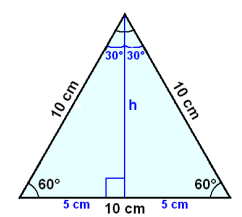We begin by drawing in the height, h, of the triangle. This divides the triangle into two equal right angled triangles. Using Pythagoras rule we can calculate h.

h2 + 52 = 102

h2 = 102 − 52 = 100 − 25 = 75 = 52×3

h = 53 ≈ 8.7

Area A  = ½×10×h ≈ ½×10×8.7 ≈ 43 cm2

### Example 4

The arms of an isosceles triangle are 30 cm in length and the base line is  42 cm. Find the length of a line drawn through the two equal sides, parallel to the base and 10 cm from the base.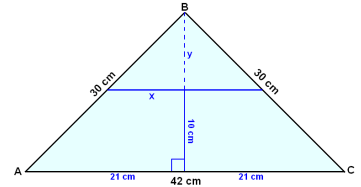First we divide the triangle into two right angled triangles by drawing in the height, h, from the vertex to the base. Now we use Pythagoras rule to calculate the height.

h2 + 212 = 302

h2 = 302 − 212 = 459

h ≈ 21.4

y = h − 10 ≈ 21.4 − 10 ≈ 11.4 cm

Using the ratio rule for similar triangles we get:

y/h = x/21

x ≈ 21×11.4/ 21.411 cm

Therefore the length of the parallel line is 22 cm.

We’ll draw the right angled triangle using the hypotenuse AB as the base line so that the angle at the vertex C is 90°. We then draw the height from C onto AB as the diagram shows: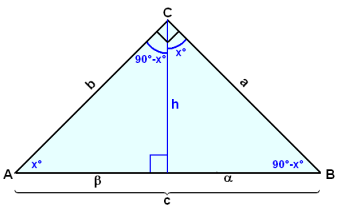This line divides the angle at the vertex into two parts ( not equal unless the triangle is isosceles). If one part is x°, then the other must be 90°− x°. We can easily see that the two base angles must be  90° − x° (on the right) and x° ( on the left ) as the sum of the angles in any triangle is always 180°.

Notice that all the angles in both the smaller triangles and also in the original triangle ABC are equal, and are  90°, x° and 90° − x°. These three triangles are therefore similar.

The following rule holds for all right angled triangles:

The height drawn from a vertex to the hypotenuse divides a right angled triangle into two triangles that are both similar to the original triangle.

This gives rise to three sets of ratios.

Using the Greek letters a for the side opposite the angle

labelled x° and b for the side opposite the angle labelled 90°− x° as shown in the diagram we get  the following:

The two smaller triangles are similar therefore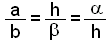The original triangle and the triangle with top angle x are similar therfore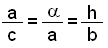The original triangle and the triangle with top angle 90°−x°  are similar therfore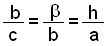### Example 5

A right angled triangle is given with the two shorter sides of length 7 cm and 10 cm. The height drawn on the hopotenuse divides the triangle into two triangles. Find the area of these two triangles.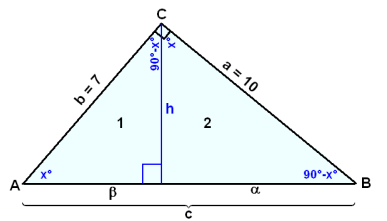First we use Pythagoras to calculate the length of the hypotenuse, c.

c2 = 102 + 72 = 149,  Then c ≈ 12.2 cm

Next we use one of the above ratios to calculate a.

a/c = a/a

a = a2/c ≈ 102/12.2 ≈ 8.2 cm

And then b.

b ≈ 12.2 − 8.2 ≈ 4 cm

Now we need to calculate the height h .

b/c = h/a

h = ab/c10×7/12.2 ≈ 5.7 cm

The areas are now easily found.

Area F1 = ½×b×h ≈ ½×4×5.7 ≈ 11.4 cm2

Area F2 = ½×a×h ≈ ½×8.2×5.7 ≈ 23.4 cm2

Try Quiz 1 on Triangles.

Remember to use the checklist to keep track of your work.